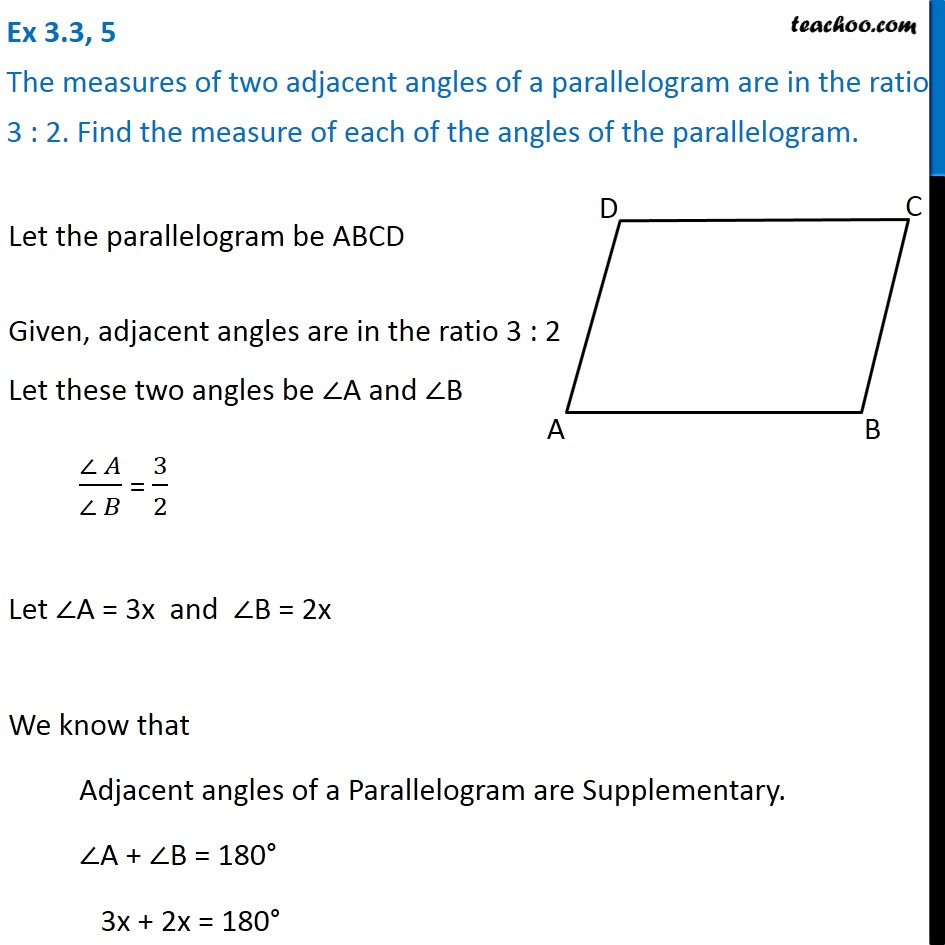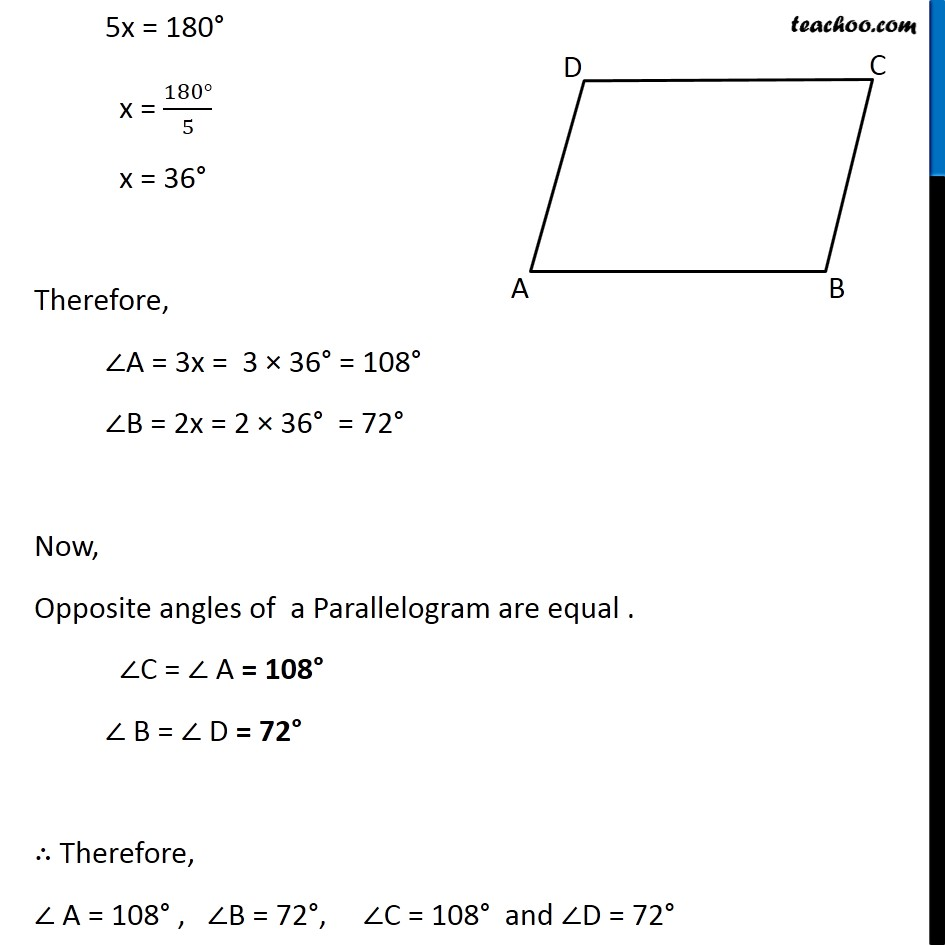Angles of Parallelogram

Chapter 3 Class 8 Understanding Quadrilaterals
Concept wiseIntroducing your new favourite teacher - Teachoo Black, at only ₹83 per month

### Transcript

Ex 3.3, 5 The measures of two adjacent angles of a parallelogram are in the ratio 3 : 2. Find the measure of each of the angles of the parallelogram.Let the parallelogram be ABCD Given, adjacent angles are in the ratio 3 : 2 Let these two angles be ∠A and ∠B (∠ 𝐴)/(∠ 𝐵) = 3/2 Let ∠A = 3x and ∠B = 2x We know that Adjacent angles of a Parallelogram are Supplementary. ∠A + ∠B = 180° 3x + 2x = 180° 5x = 180° x = (180°)/5 x = 36° Therefore, ∠A = 3x = 3 × 36° = 108° ∠B = 2x = 2 × 36° = 72° Now, Opposite angles of a Parallelogram are equal . ∠C = ∠ A = 108° ∠ B = ∠ D = 72° ∴ Therefore, ∠ A = 108° , ∠B = 72°, ∠C = 108° and ∠D = 72°